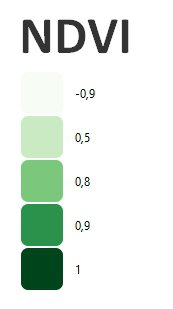What is Normalized Difference Vegetation Index (NDVI)?

The NDVI is one of the best known and most commonly used indices. It uses the red and near infrared spectral bands. The NDVI is strongly associated with vegetation content. However, this vegetation index is quite sensitive to soil brightness and atmospheric effects.

NDVI values are theoretically between -1 and +1, with negative values corresponding to surfaces other than vegetation cover, such as snow, water or clouds, for which the red reflectance is greater than the near infrared. For bare soil, reflectance in the red and near-infrared regions is roughly of the same order of magnitude, so NDVI values are close to 0. Vegetation formations, on the other hand, have positive NDVI values, generally between 0.1 and 0.7 (the highest values corresponding to the densest canopies).

How do you calculate NDVI?

It is calculated as:

NDVI = (NIR - Red) / (NIR + Red)
NDVI (Sentinel 2) = (B8 – B4) / (B8 + B4)
NDVI (Landsat 8) = (B5 – B4) / (B5 + B4)
NDVI (Landsat 4-7) = (B4 – B3) / (B4 + B3)

Example of NDVI, Rostenice farm